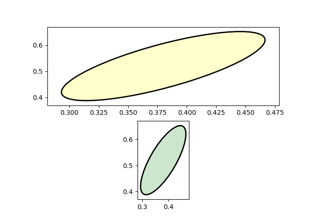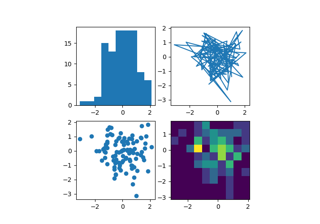# matplotlib.patches.Arc¶

class matplotlib.patches.Arc(xy, width, height, angle=0.0, theta1=0.0, theta2=360.0, **kwargs)[source]

An elliptical arc, i.e. a segment of an ellipse.

Due to internal optimizations, there are certain restrictions on using Arc:

• The arc cannot be filled.
• The arc must be used in an Axes instance. It can not be added directly to a Figure because it is optimized to only render the segments that are inside the axes bounding box with high resolution.
Parameters:
xy(float, float)

The center of the ellipse.

widthfloat

The length of the horizontal axis.

heightfloat

The length of the vertical axis.

anglefloat

Rotation of the ellipse in degrees (counterclockwise).

theta1, theta2float, default: 0, 360

Starting and ending angles of the arc in degrees. These values are relative to angle, e.g. if angle = 45 and theta1 = 90 the absolute starting angle is 135. Default theta1 = 0, theta2 = 360, i.e. a complete ellipse. The arc is drawn in the counterclockwise direction. Angles greater than or equal to 360, or smaller than 0, are represented by an equivalent angle in the range [0, 360), by taking the input value mod 360.

Other Parameters:
**kwargsPatch properties

Most Patch properties are supported as keyword arguments, with the exception of fill and facecolor because filling is not supported.

Property Description
agg_filter a filter function, which takes a (m, n, 3) float array and a dpi value, and returns a (m, n, 3) array
alpha float or None
animated bool
antialiased or aa unknown
capstyle {'butt', 'round', 'projecting'}
clip_box Bbox
clip_on bool
clip_path Patch or (Path, Transform) or None
color color
contains unknown
edgecolor or ec color or None or 'auto'
facecolor or fc color or None
figure Figure
fill bool
gid str
hatch {'/', '\', '|', '-', '+', 'x', 'o', 'O', '.', '*'}
in_layout bool
joinstyle {'miter', 'round', 'bevel'}
label object
linestyle or ls {'-', '--', '-.', ':', '', (offset, on-off-seq), ...}
linewidth or lw float or None
path_effects AbstractPathEffect
picker None or bool or callable
rasterized bool or None
sketch_params (scale: float, length: float, randomness: float)
snap bool or None
transform Transform
url str
visible bool
zorder float
__init__(self, xy, width, height, angle=0.0, theta1=0.0, theta2=360.0, **kwargs)[source]
Parameters:
xy(float, float)

The center of the ellipse.

widthfloat

The length of the horizontal axis.

heightfloat

The length of the vertical axis.

anglefloat

Rotation of the ellipse in degrees (counterclockwise).

theta1, theta2float, default: 0, 360

Starting and ending angles of the arc in degrees. These values are relative to angle, e.g. if angle = 45 and theta1 = 90 the absolute starting angle is 135. Default theta1 = 0, theta2 = 360, i.e. a complete ellipse. The arc is drawn in the counterclockwise direction. Angles greater than or equal to 360, or smaller than 0, are represented by an equivalent angle in the range [0, 360), by taking the input value mod 360.

Other Parameters:
**kwargsPatch properties

Most Patch properties are supported as keyword arguments, with the exception of fill and facecolor because filling is not supported.

Property Description
agg_filter a filter function, which takes a (m, n, 3) float array and a dpi value, and returns a (m, n, 3) array
alpha float or None
animated bool
antialiased or aa unknown
capstyle {'butt', 'round', 'projecting'}
clip_box Bbox
clip_on bool
clip_path Patch or (Path, Transform) or None
color color
contains unknown
edgecolor or ec color or None or 'auto'
facecolor or fc color or None
figure Figure
fill bool
gid str
hatch {'/', '\', '|', '-', '+', 'x', 'o', 'O', '.', '*'}
in_layout bool
joinstyle {'miter', 'round', 'bevel'}
label object
linestyle or ls {'-', '--', '-.', ':', '', (offset, on-off-seq), ...}
linewidth or lw float or None
path_effects AbstractPathEffect
picker None or bool or callable
rasterized bool or None
sketch_params (scale: float, length: float, randomness: float)
snap bool or None
transform Transform
url str
visible bool
zorder float
__module__ = 'matplotlib.patches'
__str__(self)[source]

Return str(self).

draw(self, renderer)[source]

Draw the arc to the given renderer.

Notes

Ellipses are normally drawn using an approximation that uses eight cubic Bezier splines. The error of this approximation is 1.89818e-6, according to this unverified source:

Lancaster, Don. Approximating a Circle or an Ellipse Using Four Bezier Cubic Splines.

https://www.tinaja.com/glib/ellipse4.pdf

There is a use case where very large ellipses must be drawn with very high accuracy, and it is too expensive to render the entire ellipse with enough segments (either splines or line segments). Therefore, in the case where either radius of the ellipse is large enough that the error of the spline approximation will be visible (greater than one pixel offset from the ideal), a different technique is used.

In that case, only the visible parts of the ellipse are drawn, with each visible arc using a fixed number of spline segments (8). The algorithm proceeds as follows:

1. The points where the ellipse intersects the axes bounding box are located. (This is done be performing an inverse transformation on the axes bbox such that it is relative to the unit circle -- this makes the intersection calculation much easier than doing rotated ellipse intersection directly).

This uses the "line intersecting a circle" algorithm from:

Vince, John. Geometry for Computer Graphics: Formulae, Examples & Proofs. London: Springer-Verlag, 2005.

2. The angles of each of the intersection points are calculated.

3. Proceeding counterclockwise starting in the positive x-direction, each of the visible arc-segments between the pairs of vertices are drawn using the Bezier arc approximation technique implemented in Path.arc.

## Examples using matplotlib.patches.Arc¶